The Sundial Primer created by Carl SabanskiThe Sundial Primer Index
 Wide Gnomon Equatorial Sundial Equatorial Sundial: a dial in which the dial plate is parallel to the equatorial plane and the polar-pointing gnomon is perpendicular to it. Equinoctial Sundial: another (historical) name for an equatorial sundial.Equatorial Plane: the plane through the Earth defined by the equator. In the case of typical equatorial sundial, the time is read by estimating the location of the centre of the shadow cast by the gnomon. The gnomon is usually a cylindrical rod with a small diameter. As the diameter of the rod increases it becomes more difficult to determine the centre of the shadow with any degree of accuracy.A wide cylindrical gnomon would be very useful if you wanted to build a monumental equatorial sundial. The gnomon could then be used to support the dial plate. Rather than using the centre of the gnomon's shadow, let's use one of the edges. Figure 1 shows a layout for the hour lines where the trailing edge of the shadow is used. The outer circle is divided into 24 equal parts beginning where the midnight line, located at the top, intersects the circle. A line is drawn from each of these hour points tangent to the inner circle that represents the wide gnomon. This results in hour lines with an interesting pinwheel effect.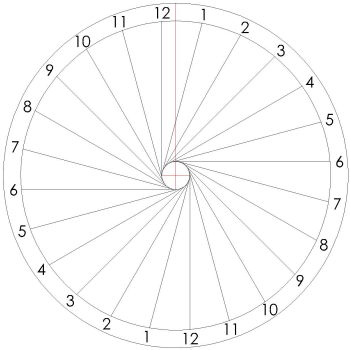Figure 1: Equatorial Sundial with Offset Hour Lines (CAD) For details regarding this design refer to Figure 2. Draw a circle at the centre of the dial plate with a diameter equal to that of the gnomon. Draw a second outer circle where the hour numbers will be placed. Draw a line from the centre of the dial vertically to the outer circle. This is the red line in the figure. Draw a line parallel to this red line but at the left edge of the gnomon. This is the midnight hour line and is a line tangent to the gnomon circle.The next step depends upon your CAD program. If it has a command for drawing polar arrays then the remaining hour lines are easy to draw. Draw a polar array, using the midnight line, for as many hour lines that are needed. The centre point of the polar array is the centre of the gnomon circle. The hour lines will be as shown in Figure 1.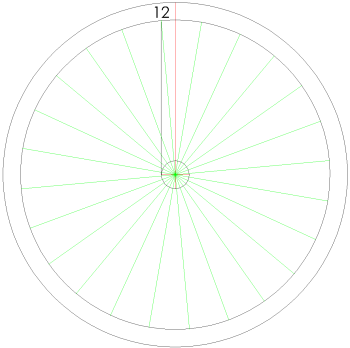Figure 2: Drawing the Tangent Hour Lines (CAD) If the polar array command is not available then draw a line from the centre of the gnomon circle to the point where the midnight line intersects the outer circle. Starting from this point divide the outer circle into as many parts as necessary for the desired number of hour lines. Each full hour line is separated by 15º. These divisions can be marked with points. If your CAD program has a command to draw a line from a point and then tangent to a circle, the hour lines can be drawn. The hour lines will be as shown in Figure 1.If drawing a line tangent to a circle is not available then draw a line from each point to the centre of the gnomon circle. These are the green lines in Figure 2. Let's look at the 4 a.m. hour line as shown in Figure 3. Draw the cyan circle with a diameter equal to the length of the green line located at 4 a.m.. The two ends of this line will lie on the circumference of the circle. This circle will cross the gnomon circle at two points. The two lines drawn from the 4 a.m. point to these two points will be tangent to the gnomon circle. However, only one of these tangent lines is the correct hour line. The correct line is shown in Figure 3. The remaining hour lines can be drawn using this same technique. The hour lines will be as shown in Figure 1.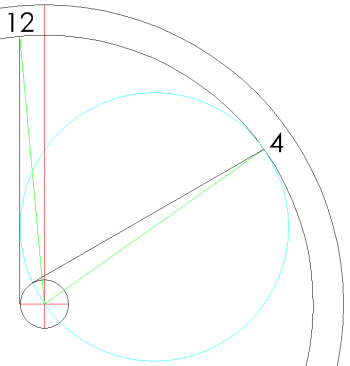Figure 3: Drawing an Hour Line Tangent to the Gnomon Circle (CAD) If the hour lines are being drawn manually then you need to find the mid-point of each green line to be able to draw the circles. The method used to do this is shown in Figure 4 and can be used for a line of any length. Draw a circular arc with the centre at one end of the green line and a radius greater than half the length of the green line. Draw a second circular arc with the centre at the other end of the green line and the same radius. These arcs, shown in cyan in Figure 4, will intersect at two points. Draw a line between these two intersection points. The point where this line crosses the green line will be the mid-point.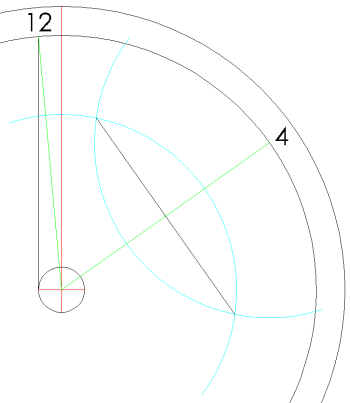Figure 4: Finding the Mid-Point of a Line (CAD) The drawing in Figure 1 is for the top plate of the equatorial sundial. The bottom plate is drawn as a mirror image along the vertical red line.The time is read from the trailing edge of the gnomon's shadow. If you want to use the leading edge of the gnomon's shadow draw the noon line to the right of the red line and then complete the remaining hour lines.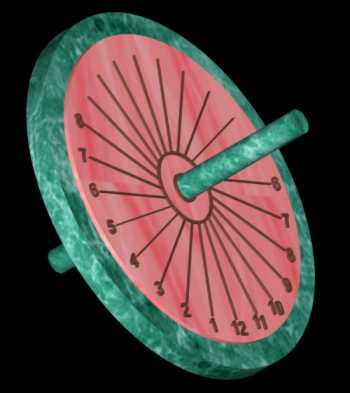For an image complete with shadow click here.